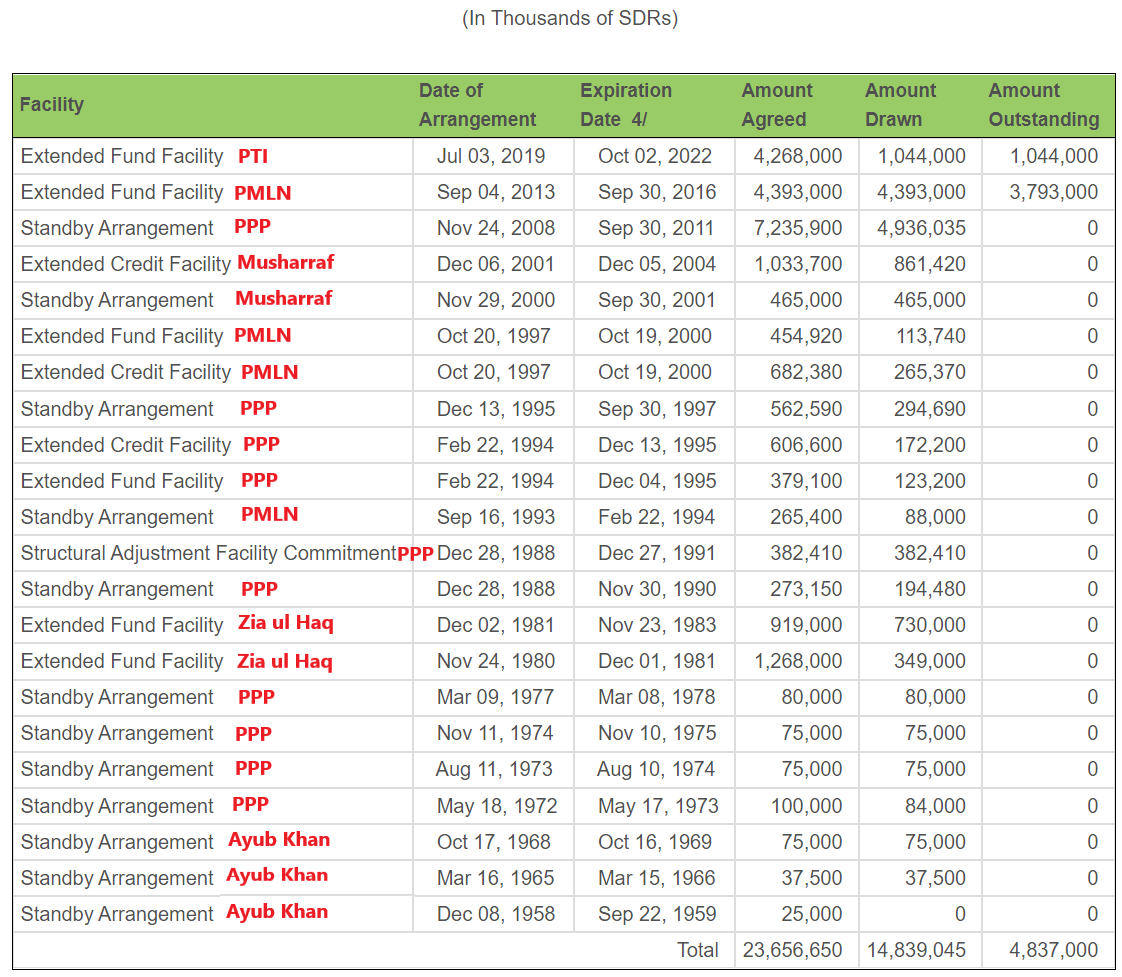# Pakistan vis-à-vis the IMFPhoto Source: IMF History of Loans to Pakistan
• 54.5 percent of commitments (12) had an expiration of 1 year.
• 18.2 percent of commitments (04) had an expiration of 2 years.
• 27.3 percent of commitments (06) had an expiration of 3 years.
• Dec 08, 1958: The SDR rate was \$1.00. Amount: 0.
• Mar 16, 1965: The SDR rate was \$1.00. Amount: \$0.037 billion.
• Oct 17, 1968: The SDR rate was \$1.00. Amount: \$0.075 billion.
• May 18, 1972: The SDR rate was \$1.00. Amount: \$0.084 billion.
• Aug 11, 1973: The assumed SDR rate is \$1.00. Amount: \$0.075 billion.
• Nov 11, 1974: The assumed SDR rate is \$1.00. Amount: \$0.075 billion.
• Mar 09, 1977: The assumed SDR rate is \$1.00. Amount: \$0.080 billion.
• Nov 24, 1980: The assumed SDR rate is \$1.00. Amount: \$0.349 billion.
• Dec 02, 1981: The SDR rate was \$1.17. Amount: \$0.854 billion.
• Dec 28, 1988: The SDR rate was \$1.34. Amount: \$0.260 billion.
• Dec 28, 1988: The SDR rate was \$1.34. Amount: \$0.512 billion
• Sep 16, 1993: The SDR rate was \$1.43. Amount: \$0.126 billion.
• Feb 22, 1994: The SDR rate was \$1.40. Amount: \$0.172 billion.
• Feb 22, 1994: The SDR rate was \$1.40. Amount: \$0.241 billion.
• Dec 13, 1995: The SDR rate was \$1.48. Amount: \$0.436 billion.
• Oct 20, 1997: The SDR rate was \$1.36. Amount: \$0.361 billion.
• Oct 20, 1997: The SDR rate was \$1.36. Amount: \$0.154 billion.
• Nov 29, 2000: The SDR rate was \$1.28. Amount: \$0.595 billion.
• Dec 06, 2001: The SDR rate was \$1.26. Amount: \$1.085 billion.
• Nov 24, 2008: The SDR rate was \$1.48. Amount: \$7.305 billion.
• Sep 04, 2013: The SDR rate was \$1.51. Amount: \$6.633 billion.
• Jul 03, 2019: The SDR rate was \$1.38. Amount: \$1.440 billion.
• The Ayub Khan-led government took IMF loans on two (02) occasions worth \$0.112 billion from 1958 to 1968. It constitutes a meager 0.54 percent share of the total IMF loans.
• The Zia-ul-Haq-led regime took IMF loans on two (02) occasions worth \$1.203 billion from 1980 to 1981. It constitutes a 5.74 percent share of the total IMF loans.
• The PMLN government took IMF loans on four (04) occasions worth \$7.274 billion: \$0.126 billion in 1993, \$0.515 billion in 1997, and \$6.633 billion in 2013. It constitutes another significant 34.72 percent share of the total IMF loans.
• The Musharraf-led regime took IMF loans on two (02) occasions worth \$1.680 billion from 2000 to 2001. It constitutes an 8.02 percent share of the total IMF loans.
• The PTI government took IMF loans on a single occasion worth \$1.440 billion in 2019. It constitutes a 6.87 percent share of the total IMF loans.